# Controlling Conducted EMI when Using Long Output Lines (Part I)Get valuable resources straight to your inbox - sent out once per month

In many automotive electronics applications, the load must be connected to the motherboard via a long output line, resulting in excessive conducted EMI. Automotive applications that require long-line loads include Class-D power amplifiers, LEDs, and USB chargers (see Figure 1).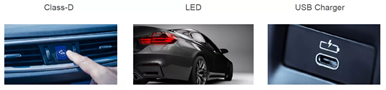Figure 1: Automotive Applications

This article is the first part of a three-part series exploring methods to analyze and improve excessive conducted EMI under long-line loads. Part I will cover the conducted EMI test results of a long-term load, as well as an analytical framework using the common-mode (CM) EMI model. Part II will analyze the transmission line effect on the output long-line impedance to ground. Part III will review three EMI noise reduction methods to analyze and predict resonant peaks.

## Long-Term Load EMI Test Device

When testing for conducted EMI, ensure that the output line’s length is consistent with the actual application. Figure 2 shows the test device of a long-term load. This load is based on the CISPR 25 standard, which protects on-board vehicle receivers. The equipment under test (EUT) is placed 5cm away from the reference ground (copper plate), and a low dielectric constant (with a relative dielectric constant below 1.4) serves as the medium. The output line is about 1m to 2m depending on the actual application. LISN is connected between the power supply and EUT to provide a constant impedance for the noise loop. The impedance is 25Ω in standard mode.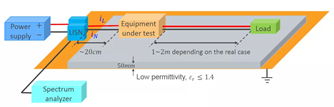Figure 2: Long-Term Load Test Device Based on CISPR 25

Figure 3 shows a comparison between the conducted EMI test results of the on-board vehicle Class-D power amplifier (2.2MHz, bridge-tied load, 24.5W, analog input) without an output cable and with a 2m output cable. In the absence of an output cable, the Class-D power amplifier’s EMI can meet the CISPR 25 requirements. With a 2m output cable, the EMI spikes violate CISPR 25, especially at the 30MHz and 90MHz peaks.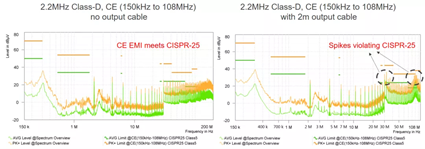Figure 3: Long-Term Load Comparison (No Output Cable and 2m Output Cable)

To address the EMI spikes during long-term loads, MPS uses a CM EMI model to interpret the conducted EMI test results. These results are later tested using a transmission line model and noise reduction methods.

## CM EMI Model

Consider a Class-D topology and its conducted CM noise path (see Figure 4). The dV/dt node and the switching frequency’s dI/dt loop in the circuit generates the CM noise. The noise passes through the output filter to the output side, then through the output side to the ground impedance at the reference ground. Lastly, CM noise flows from LISN back to the EUT. The output cable’s parasitic impedance (ZP) to ground is important for the conducted EMI analysis. Additionally, the dV/dt node’s parasitic capacitance (CSWP) provides a path for CM noise to the reference ground.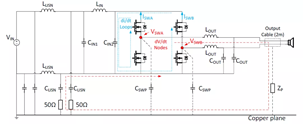Figure 4: CM Noise Path of Class-D Power Amplifier

According to the substitution theorem, when analyzing EMI, a voltage source or a current source can substitute the voltage or current on the switch. Figure 5 shows the circuit diagram after applying the substitution theorem.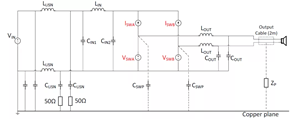Figure 5: Current Diagram with the Substitution Theorem

Next, apply the superposition theorem to individually analyze the noise generated by each source. Unlike the voltage source, the current source does not generate noise by itself. Figure 6 shows how the the common-noise current source is analyzed based on the superposition theorem.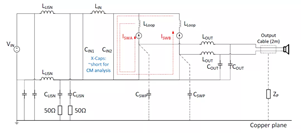Figure 6: Analysis of CM Noise Current Source

Figure 7 shows the analysis of the CM noise voltage source based on the superposition theorem, which also yields the preliminary CM model.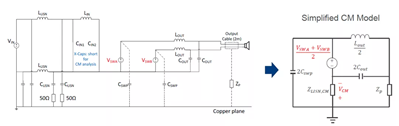Figure 7: Analysis of the Common-Mode Noise Voltage Source

It is necessary to also consider near-field coupling between the output line and EUT, since the output line is a large conductor. There are two types of near-field coupling: electric field coupling and magnetic field coupling.

Electric field coupling refers to a circuit where a parasitic capacitor is placed between one conductor (the first switching node (SWA) and second switching node (SWB)) and another conductor (an output line). Figure 8 shows that if the conductor is a high-frequency dV/dt node, current noise flows to the second and generates EMI noise.

Magnetic field coupling refers to the inductance between one loop (the loop between the switch and the input capacitor) and a second loop (the loop between the output line and the reference ground). Figure 8 shows that if one loop is a high-frequency dI/dt loop, the other loop generates an induced electromotive force, resulting in EMI noise.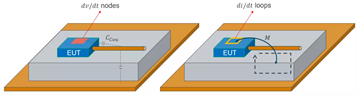Figure 8: EMI Noise Generated by Electric Field Coupling and Magnetic Field Coupling

For electric field coupling, the simplified CM model from Figure 7 can be modified. Figure 9 shows that CCOU represents the capacitor coupled from the switch node to the output line. At high frequencies, the capacitor’s impedance is minimal and bypasses the current noise to generate EMI.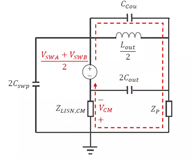Figure 9: Modified EMI Model for Electric Field Coupling

The dI/dt loop from Figure 6 can be modified to generate magnetic field coupling. Figure 10 shows how the CM path gains an additional noise source after decoupling. The noise is proportional to dI/dt, as well as the inductance between the input loop and the output line-to-ground loop.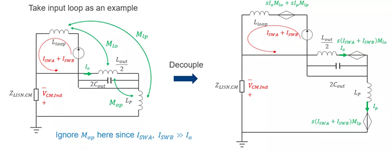Figure 10: Modified EMI Model for Magnetic Field Coupling

## Summary

In this article, we reviewed the test device’s long-term load conducted EMI results, and applied the substitution and superposition theorems to develop the CM EMI model. We also considered the problem of near-field coupling, which can be broadly categorized between electric field coupling and magnetic field coupling. Part II will go further in depth with the transmission line model to obtain the output long-line impedance to ground. Part III will review three EMI noise reduction methods to analyze and predict resonant peaks.

### Did you find this interesting? Get valuable resources straight to your inbox - sent out once per month!

Get technical support